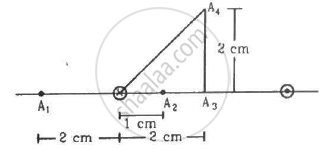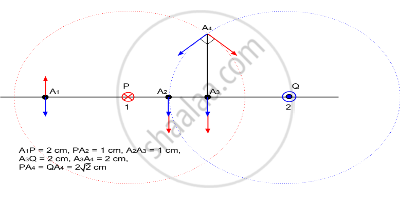Advertisement Remove all ads

# Figure Shows Two Parallel Wires Separated by a Distance of 4.0 Cm and Carrying Equal Currents of 10 a Along Opposite Directions. Find the Magnitude of the Magnetic Field B at the Points A1, A2, A3. - Physics

Short Note

Figure shows two parallel wires separated by a distance of 4.0 cm and carrying equal currents of 10 A along opposite directions. Find the magnitude of the magnetic field B at the points A1, A2, A3Advertisement Remove all ads

#### Solution

For point A1,
Magnitude of current in wires, I = 10 A
Separation of point A1 from the wire on the left side, d = 2 cm
Separation of point A1 from the wire on the right side, d' = 6 cmIn the figure
Red and blue arrow denotes the direction of magnetic field due to the wire marked as red and blue respectively.
P (marked red) denotes the wire carrying current in a plane going into the paper.
Q (marked blue) denotes the wire carrying current in a plane coming out of the paper.
Also from the figure, we can see that

$P A_4 = Q A_4$

$\angle A_4 A_3 P = \angle A_4 A_3 Q = 90^\circ$

$\Rightarrow \angle A_4 P A_3 = \angle A_4 Q A_3 = 45^\circ$

$\Rightarrow \angle P A_4 A_3 = \angle Q A_4 A_3 = 45^\circ$

$\Rightarrow \angle P A_4 Q = 90^\circ$

The magnetic field at A1 due to current in the wires is given by

$B = \frac{\mu_0 I}{2\pi d} - \frac{\mu_0 I}{2\pi d'}$    ...(1)

$\Rightarrow B = \frac{2 \times {10}^{- 7} \times 10}{2 \times {10}^{- 2}} - \frac{2 \times {10}^{- 7} \times 10}{6 \times {10}^{- 2}}$

$= \left( 1 - \frac{1}{3} \right) \times {10}^{- 4}$

$= 0 . 67 \times {10}^{- 4} T$

Similarly, we get the magnetic field at A2 using eq. (1).

$B = \frac{2 \times {10}^{- 7} \times 10}{1 \times {10}^{- 2}} + \frac{2 \times {10}^{- 7} \times 10}{3 \times {10}^{- 2}}$

$= \frac{8}{3} \times {10}^{- 4} T$

$= 2 . 67 \times {10}^{- 4} T$

Now,
Magnetic field at A3:

$B = \frac{2 \times {10}^{- 7} \times 10}{2 \times {10}^{- 2}} + \frac{2 \times {10}^{- 7} \times 10}{2 \times {10}^{- 2}}$

$= 2 \times {10}^{- 4} T$

Magnetic field at A4:
Separation of point A4 from the wire on the left side, d = $\sqrt{2^2 + 2^2} = 2\sqrt{2}$ cm
Separation of point A4 from the wire on the right side, d' =$\sqrt{2^2 + 2^2} = 2\sqrt{2}$ cm
Thus, the magnetic field at A4 due to current in the wires is given by

$B = \sqrt{\left( \frac{2 \times {10}^{- 7} \times 10}{2\sqrt{2} \times {10}^{- 2}} \right)^2 + \left( \frac{2 \times {10}^{- 7} \times 10}{2\sqrt{2} \times {10}^{- 2}} \right)^2}$

$= 1 \times {10}^{- 4} T$

Is there an error in this question or solution?
Advertisement Remove all ads

#### APPEARS IN

HC Verma Class 11, Class 12 Concepts of Physics Vol. 2
Chapter 13 Magnetic Field due to a Current
Q 9 | Page 250
Advertisement Remove all ads

#### Video TutorialsVIEW ALL 

Advertisement Remove all ads
Share
Notifications

View all notifications

Forgot password?